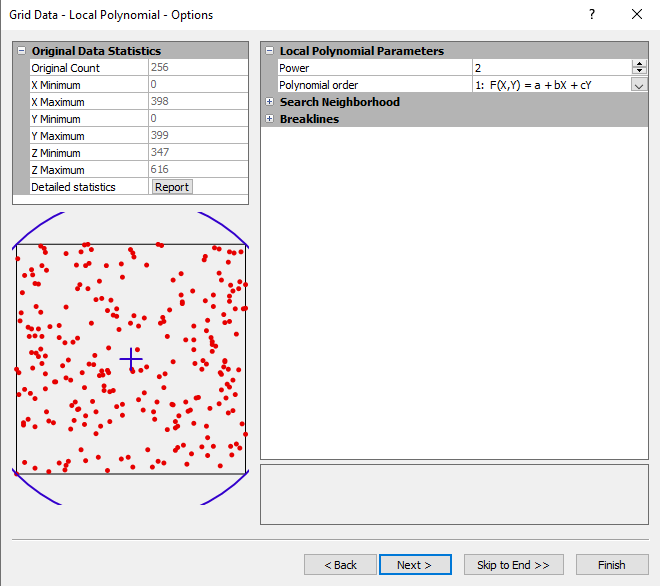# Local Polynomial

The Local Polynomial gridding method assigns values to grid nodes by using a weighted least squares fit with data within the grid node's search ellipse.

## Local Polynomial Options Dialog

In the Grid Data dialog, specify Local Polynomial as the Gridding Method and click the Next button to open the Grid Data Local Polynomial Options dialog.Choose a Power and Polynomial Order in the Grid Data Local Polynomial Options dialog.

### Power

Set the Power to a number between 0 and 20. The Power parameter is used to assign the power for the weighted least square function, see Local Polynomial Math. This allows data closer to the grid node to have more impact on the value of the grid node than data farther away. This parameter is similar to the Inverse Distance to a Power gridding method.

### Polynomial Order

Select a Polynomial Order, 1, 2, or 3.

1: F(X,Y) = a +bX + cY

2: F(X,Y) = a +bX + cY + dXY + eX2 + fX2

3: F(X,Y) = a +bX + cY + dXY + eX2 + fX2 +gX2Y + hXY2 + iX3 + iY3